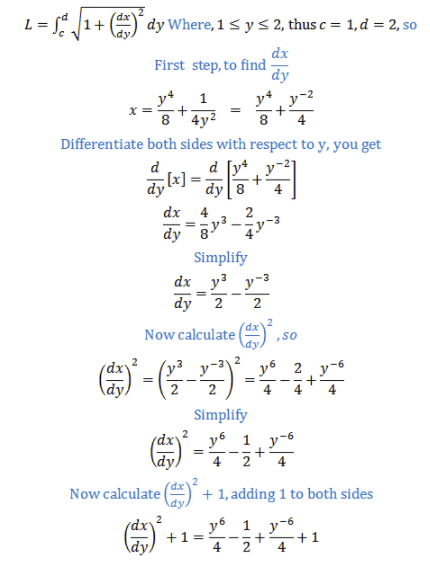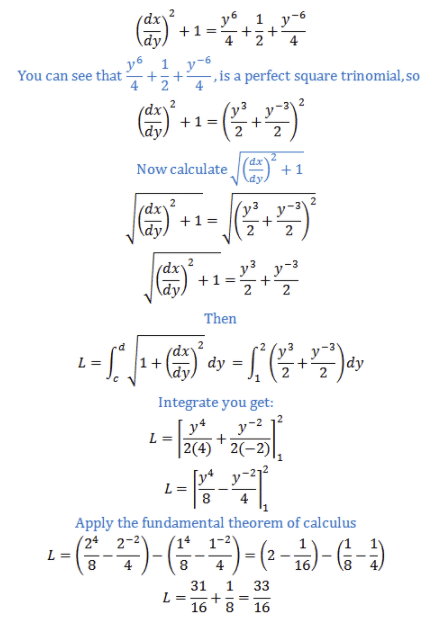# Find The Exact Length of The Curve. X = y4 8 + 1 4y2 , 1 ≤ y ≤ 2

We thoroughly check each answer to a question to provide you with the most correct answers. Found a mistake? Let us know about it through the REPORT button at the bottom of the page.

Find the exact length of the curve. x=y^4/8+1/4y^2, 1<=y<=2

Contents

You need to apply the next formula:## Result

L = 33/16